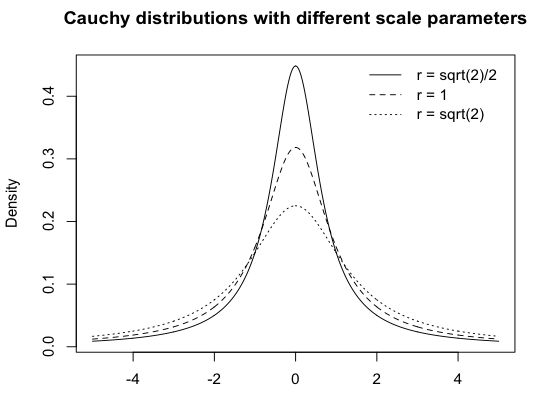# Interactive exploration of a prior’s impact

The probably most frequent criticism of Bayesian statistics sounds something like “It’s all subjective – with the ‘right’ prior, you can get any result you want.”.
In order to approach this criticism it has been suggested to do a sensitivity analysis (or robustness analysis), that demonstrates how the choice of priors affects the conclusions drawn from the Bayesian analysis. Ideally, it can be shown that for virtually any reasonable prior the conclusions remain the same. In this case, the critique “it’s all in the prior” can be refuted on empirical grounds.
In their recent paper “The p < .05 rule and the hidden costs of the free lunch in inference” Jeff Rouder and colleagues argue that in the case of the default Bayes factor for t tests the choice of the H1 prior distribution does not make a strong difference (see Figure 6, right panel). They come to the conclusion that “Prior scale does matter, and may change the Bayes factor by a factor of 2 or so, but it does not change the order of magnitude.” (p. 24).
The default Bayes factor for t tests (Rouder, Speckman, Sun, Morey, Iverson, 2009) assumes that effect sizes (expressed as Cohen’s d) are distributed as a Cauchy distribution (this is the prior distribution for H1). The spread of the Cauchy distribution can be changed with the scale parameter r. Depending on the specific research area, one can use a wider (large r‘s, e.g. r =1.5) or a thinner (small r’s, e.g. r = 0.5) Cauchy distribution. This corresponds to the prior belief that typically larger or smaller effect sizes can be expected.
For the two-sample t test, the BayesFactor package for R suggest three defaults for the scale parameter:

• “medium” (r = sqrt(2)/2 = 0.71),
• “wide” (r = 1), and
• “ultra-wide” (r = sqrt(2) = 1.41).

Here’s a display for these distributions:For a given effect size: How does the choice of the prior distribution change the resulting Bayes factor?
The following shiny app demonstrates how the choice of the prior influences the Bayes factor for a given effect size and sample size. Try moving the sliders! You can also provide arbitrary values for r (as comma-separated values; r must be > 0; reasonable ranges are between 0.2 and 2).
For a robustness analysis simply compare the lines at each vertical cut. An important line is the solid blue line at log(1), which indicates the same support for H1 and H0. All values above that line are in favor of the H1, all values below that line are in favor of H0.

As you will see, in most situations the Bayes factors for all r‘s are either above log(1), or below log(1). That means, regardless of the choice of the prior you will come to the same conclusion. There are very few cases where a data line for one r is below log(1) and the other is above log(1).  In this case, different r‘s would come to different conclusions. But in these ambiguous situations the evidence for H1 or for H0 is always in the “anectodal” region, which is a very weak evidence. With the default r’s, the ratio of the resulting Bayes factors is indeed maximally “a factor of 2 or so”.
To summarize, within a reasonable range of prior distributions it is not possible that one prior generates strong evidence for H1, while some other prior generates strong evidence for H0. In that sense, the conclusions drawn from a default Bayes factor are robust to the choice of (reasonable) priors.

### References

Rouder, J. N., Morey, R. D., Verhagen, J., Province, J. M., & Wagenmakers, E. J. (submitted). The p < .05 rule and the hidden costs of the free lunch in inference. Retrieved from http://pcl.missouri.edu/biblio/author/29
Rouder, J. N., Speckman, P. L., Sun, D., Morey, R. D., & Iverson, G. (2009). Bayesian t tests for accepting and rejecting the null hypothesis. Psychonomic Bulletin & Review, 16, 225–237.

## 3 thoughts on “Interactive exploration of a prior’s impact”

1.Richard D. Morey says:

I discussed this issue on the BayesFactor blog as well; see my post here. You might also be interested in the shiny app I created for the end of that post, which complements yours.

2.Lettie says:

great points altogether, you simply received a emblem new